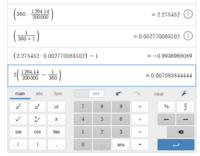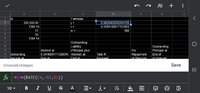# Newton-Raphson method

#### NewtonsEye

##### New member
Hello All,
Attempting to use Newton-Raphson method to determine APR. According to my workbook, it says:

The first guess for using Newton-Raphson method to solve for i must be to the right of the critical point, where the curve has a derivative of zero (any estimate to the left of the critical point results in a false solution of 0.000%)."

That critical point occurs at:
ilow = (Nx/P)(1/(N + 1)) – 1
I CANNOT PRODUCE THE 0.002279791 ANSWER. I ALWAYS GET -0.9936968089 (see calculator print below). NOT SURE IF THIS FORMULA IS WRONG OR PERHAPS MY SETUP???

A useful upper bound (although higher estimates still converge), is the case where the interest is not compounded: ihigh = 2(x/P - 1/N) - WORKS FINE

The equation in this case for finding successive estimates for i is:

i' = i - (P*i - x(1 - R - N) / (P – Nx / R(N + 1))
Or
i' = x(RN - 1 - Ni/R) / (P *R^N – Nx/R) - ALSO CANNOT DUPLICATE THE RESULTS SHOWN FOR i1-i4.

Example:

Use the following to solve for i:
P = $200,000.00 x =$1,264.14
N = 360 (months)
R = 1 + i
V = 1/R (periodic discount factor)

The theoretical low is 0.002279791 (APR = 2.736%)
- I GET -0.993696808
The theoretical high is 0.0070858441 (APR = 8.503%) - WORKS FINE

Make the theoretical high as the first guess, and continue to refine i using the Newton-Raphson derived equation for i' until it converges:
i0 = 0.007085844 (APR = 8.503%)
i1 = 0.005550004 (APR = 6.660%)
i2 = 0.005418138 (APR = 6.502%)
i3 = 0.005416692 (APR = 6.500%)
i4 = 0.005416692 (APR = 6.500%)

Since i4 = i3, we have converged on the non-zero root for the equation, and the correct APR is 6.500%.
In the context of solving for the periodic interest rate i, we used the notation i4 to represent the result of fourth iteration of the Newton-Raphson method.Any insights would be much appreciated!
Newt

#### jonah2.0

##### Full Member
Beer soaked ramblings follow.
Hello All,
Attempting to use Newton-Raphson method to determine APR. According to my workbook, it says:

The first guess for using Newton-Raphson method to solve for i must be to the right of the critical point, where the curve has a derivative of zero (any estimate to the left of the critical point results in a false solution of 0.000%)."

That critical point occurs at:
ilow = (Nx/P)(1/(N + 1)) – 1
I CANNOT PRODUCE THE 0.002279791 ANSWER. I ALWAYS GET -0.9936968089 (see calculator print below). NOT SURE IF THIS FORMULA IS WRONG OR PERHAPS MY SETUP???

A useful upper bound (although higher estimates still converge), is the case where the interest is not compounded: ihigh = 2(x/P - 1/N) - WORKS FINE

The equation in this case for finding successive estimates for i is:

i' = i - (P*i - x(1 - R - N) / (P – Nx / R(N + 1))
Or
i' = x(RN - 1 - Ni/R) / (P *R^N – Nx/R) - ALSO CANNOT DUPLICATE THE RESULTS SHOWN FOR i1-i4.

Example:

Use the following to solve for i:
P = $200,000.00 x =$1,264.14
N = 360 (months)
R = 1 + i
V = 1/R (periodic discount factor)

The theoretical low is 0.002279791 (APR = 2.736%)
- I GET -0.993696808
The theoretical high is 0.0070858441 (APR = 8.503%) - WORKS FINE

Make the theoretical high as the first guess, and continue to refine i using the Newton-Raphson derived equation for i' until it converges:
i0 = 0.007085844 (APR = 8.503%)
i1 = 0.005550004 (APR = 6.660%)
i2 = 0.005418138 (APR = 6.502%)
i3 = 0.005416692 (APR = 6.500%)
i4 = 0.005416692 (APR = 6.500%)

Since i4 = i3, we have converged on the non-zero root for the equation, and the correct APR is 6.500%.
In the context of solving for the periodic interest rate i, we used the notation i4 to represent the result of fourth iteration of the Newton-Raphson method.

View attachment 26313

Any insights would be much appreciated!
Newt
You could also use Excel or Google's Sheets app.•Subhotosh Khan

#### NewtonsEye

##### New member
Thank you Jonah!
Good tips on how to solve for i, but I'm specifically trying to illustrate the use of the Newton-Raphson method, and document the correct formula for the first (low) guess.

In using this method, you must always make your first (low) guess to the right of the critical point, where the curve has a derivative of zero, as any estimate to the left of the critical point will result in a false solution of 0.000%. That critical point can be calculated by using the formula:
ilow = (Nx/P)(1/(N + 1)) – 1
.

But in this example, the formula is supposed to yield an answer of 0.002279791, which I cannot duplicate.

I think there might be a notation error in the shown formula, but so far, I haven't been able to figure out how it's wrong.

#### Subhotosh Khan

##### Super Moderator
Staff member
Thank you Jonah!
Good tips on how to solve for i, but I'm specifically trying to illustrate the use of the Newton-Raphson method, and document the correct formula for the first (low) guess.

In using this method, you must always make your first (low) guess to the right of the critical point, where the curve has a derivative of zero, as any estimate to the left of the critical point will result in a false solution of 0.000%. That critical point can be calculated by using the formula:
ilow = (Nx/P)(1/(N + 1)) – 1
.

But in this example, the formula is supposed to yield an answer of 0.002279791, which I cannot duplicate.

I think there might be a notation error in the shown formula, but so far, I haven't been able to figure out how it's wrong.
If you want us to determine "mistake" in your calculation:

Please write the complete functions that you need to solve for its roots.​
Please include nominal description of all the variables along with the dimensions (units) of those variables.​